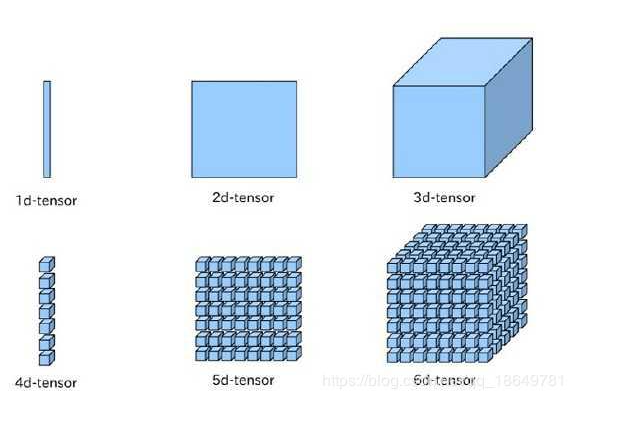WHCSRL 技术网

# 学习记录1-python图片分割&transforms方法_wzx

## python 图片分割

``````import os
from PIL import Image
Image.MAX_IMAGE_PIXELS = None

def splitimage(src, rownum, colnum, dstpath):
img = Image.open(src)
w, h = img.size
if rownum <= h and colnum <= w:
print('Original image info: %%sx%%s, %%s, %%s' %% (w, h, img.format, img.mode))
print('开始处理图片切割, 请稍候...')

s = os.path.split(src)
if dstpath == '':
dstpath = s
fn = s.split('.')
basename = fn
ext = fn[-1]

num = 0
rowheight = h // rownum
colwidth = w // colnum
for r in range(rownum):
for c in range(colnum):
box = (c * colwidth, r * rowheight, (c + 1) * colwidth, (r + 1) * rowheight)
img.crop(box).save(os.path.join(dstpath, basename + '_' + str(num) + '.' + ext), ext)
num = num + 1

print('图片切割完毕，共生成 %%s 张小图片。' %% num)
else:
print('不合法的行列切割参数！')

src = input('请输入图片文件路径：')
if os.path.isfile(src):
dstpath = input('请输入图片输出目录（不输入路径则表示使用源图片所在目录）：')
if (dstpath == '') or os.path.exists(dstpath):
row = int(input('请输入切割行数：'))
col = int(input('请输入切割列数：'))
if row > 0 and col > 0:
splitimage(src, row, col, dstpath)
else:
print('无效的行列切割参数！')
else:
print('图片输出目录 %%s 不存在！' %% dstpath)
else:
print('图片文件 %%s 不存在！' %% src)

```12345678910111213141516171819202122232425262728293031323334353637383940414243444546```

## transforms方法,常用的数据预处理方法，提高泛化能力

1.resize:transforms.Resize

size:- If size is an int, if height > width, then image will be rescaled to (size * height / width, size)，所以建议size设定为h*w
interpolation-插值方法选择
2.中心裁剪：transforms.CenterCrop

size-(sequence or int ),若为sequence，则为（和，w）,若为int，则（size,size）
3.transforms.ToTensorrank就是上文所说的阶。他是指这个张量拥有的基底向量的数量，也就是他的方向的数量。从上图中其实能明白为什么是一阶，二阶，三阶。

rank=1: [0,1,2]

rank=2: [[0,1],[2,3]]

rank=3: [[[0,1],[2,3]],[[4,5],[6,7]]]

shape是形状，他指明每一层有多少个元素。比如[2,3,4]是指第一层2个元素，第二层3个元素，第三层4个元素，通过这个我们就可以知道这个张量一共有2 × 3 × 4=24 个元素。

4.数据归一化处理transforms.Normalize